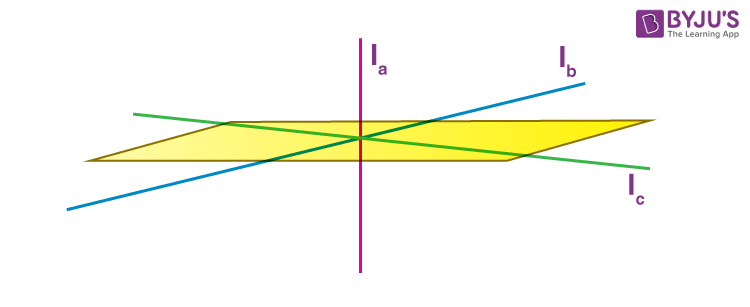# Parallel & Perpendicular Axis Theorems

## What is Parallel Axis Theorem?

The parallel axis theorem states that

The moment of inertia of a body about an axis parallel to the body passing through its centre is equal to the sum of the moment of inertia of the body about the axis passing through the centre and the product of the mass of the body times the square of the distance of between the two axes.

## Parallel Axis Theorem Formula

Parallel axis theorem statement can be expressed as follows:

 I = Ic + Mh2

Where,

• I is the moment of inertia of the body
• Ic is the moment of inertia about the center
• M is the mass of the body
• h2 is the square of the distance between the two axes

## Parallel Axis Theorem Derivation

Let Ic be the moment of inertia of an axis that is passing through the centre of mass (AB from the figure) and I will be the moment of inertia about the axis A’B’ at a distance of h.

Consider a particle of mass m at a distance r from the centre of gravity of the body.
Then,
Distance from A’B’ = r + h

I = ∑m (r + h)2

I = ∑m (r2 + h2 + 2rh)

I = ∑mr2 + ∑mh2 + ∑2rh

I = Ic + h2∑m + 2h∑mr

I = Ic + Mh2 + 0

I = Ic + Mh2

Hence, the above is the formula of parallel axis theorem.### Parallel Axis Theorem of Rod

The parallel axis theorem of rod can be determined by finding the moment of inertia of rod.
Moment of inertia of rod is given as:

$$\begin{array}{l} I = \frac{1}{3} ML^2\end{array}$$

The distance between the end of the rod and its centre is given as:

$$\begin{array}{l}h = \frac{L}{2}\end{array}$$

Therefore, the parallel axis theorem of the rod is:

$$\begin{array}{l}I_c = \frac{1}{3} ML^2 – M(\frac{L}{2})^2\end{array}$$

$$\begin{array}{l}I_{c} = \frac{1}{3} ML^2 – \frac{1}{4}ML^2\end{array}$$
$$\begin{array}{l}I_c = \frac{1}{12} ML^2 \end{array}$$

## From the video, learn to solve problems based on parallel axis theorem## Perpendicular Axis Theorem

The perpendicular axis theorem states that

For any plane body, the moment of inertia about any of its axes which are perpendicular to the plane is equal to the sum of the moment of inertia about any two perpendicular axes in the plane of the body which intersect the first axis in the plane.

## Perpendicular Axis Theorem Formula

Perpendicular axis theorem is used when the body is symmetric in shape about two out of the three axes. If the moment of inertia about two of the axes are known the moment of inertia about the third axis can be found using the expression:

 $$\begin{array}{l}I_a = I_b+I_c\end{array}$$Say in an engineering application, we have to find the moment of inertia of a body, but the body is irregularly shaped, and the moment of in these cases, we can make use of the parallel axis theorem to get the moment of inertia at any point as long as we know the centre of gravity of the body. This is a very useful theorem in space physics, where the calculation of the moment of inertia of spacecraft and satellites makes it possible for us to reach the outer planets and even the deep space. The theorem of the perpendicular axis helps in applications where we don’t have access to one axis of a body, and it is vital for us to calculate the moment of inertia of the body in that axis.

Assignment: If the moment of inertia of a body along a perpendicular axis passing through its centre of gravity is 50 kg·m2 and the mass of the body is 30 Kg. What is the moment of inertia of the same body along another axis, which is 50 cm away from the current axis and parallel to it?

Solution: From the parallel axis theorem,

$$\begin{array}{l} I = I_G + Mb^2 \end{array}$$

$$\begin{array}{l}I = 50 + ( 30 \times 0.5^2 )\end{array}$$

$$\begin{array}{l}I = 57.5\,kg-m^2\end{array}$$

### Recommended Video## Frequently Asked Questions – FAQs

Q1

### When to use parallel axis theorem?

The parallel axis theorem is used for finding the moment of inertia of the area of a rigid body whose axis is parallel to the axis of the known moment body, and it is through the centre of gravity of the object.

Q2

### What are the applications of perpendicular axis theorem?

Following are the applications of perpendicular axis theorem:

• The calculation of the moment of inertia about the third axis is possible with the help of the perpendicular axis theorem.
• The moment of inertia for three-dimensional objects can be determined by using the perpendicular axis theorem.
Q3

### What is the relation between the H – vertical axis, I – a moment of inertia, and K – radius of gyration in terms of perpendicular axes?

The relation between H – vertical axis, I – a moment of inertia, and K – radius of gyration in terms of perpendicular axes is Izz = Ixx + Iyy.

Q4

### What is the relevance of distance in parallel axis theorem?

In the parallel axis theorem, two mutually perpendicular moments of inertia is used for the calculation of the area. This is determined by using the square of the distance from the axis of rotation.

Q5

### What is radius of gyration?

The radius of gyration is defined as the distance of a point where the whole area of the body is assumed to be concentrated from the given axis.

Test your Knowledge on Parallel Perpendicular Axes Theorem